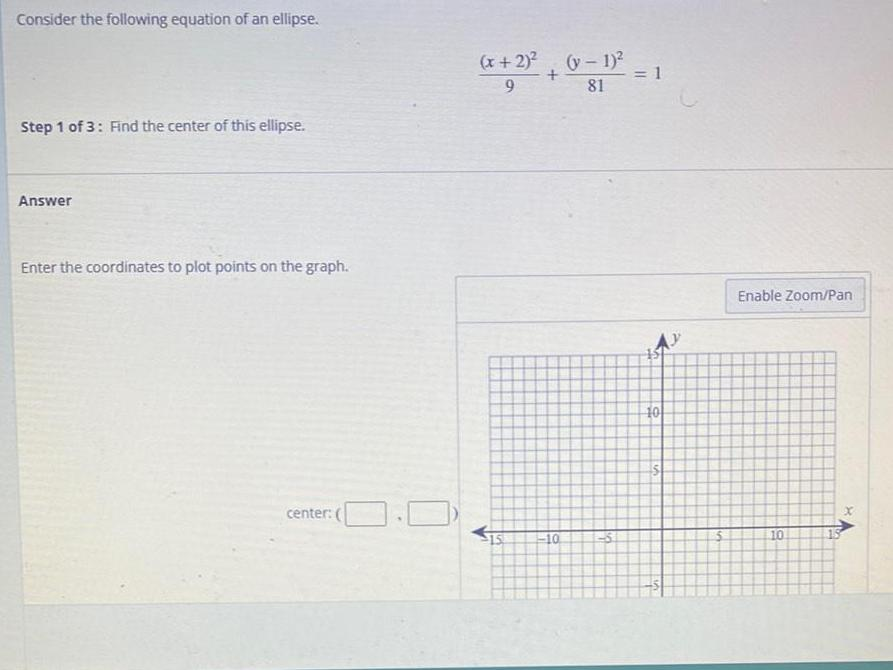Question:

# Consider the following equation of an ellipse. (x+2)^2 /9 +

Last updated: 8/12/2022Consider the following equation of an ellipse. (x+2)^2 /9 + (y-1)^2 /81 =1 Find the center of this ellipse. Answer Enter the coordinates to plot points on the graph. center: